• ## Latex画图

千次阅读 2019-11-05 20:52:08
第一次正式用latex画图，讲道理代码挺粗糙的，估计有简化的方法，然而我不会啊。 暂时有两个改进点 如何批量修改图中字体大小 如何批量修改线段大小 图片 代码 \documentclass[a4paper]{article} \usepackage{...
介绍
第一次正式用latex画图，讲道理代码挺粗糙的，估计有简化的方法，然而我不会啊。
暂时有两个改进点

如何批量修改图中字体大小
如何批量修改线段大小

图片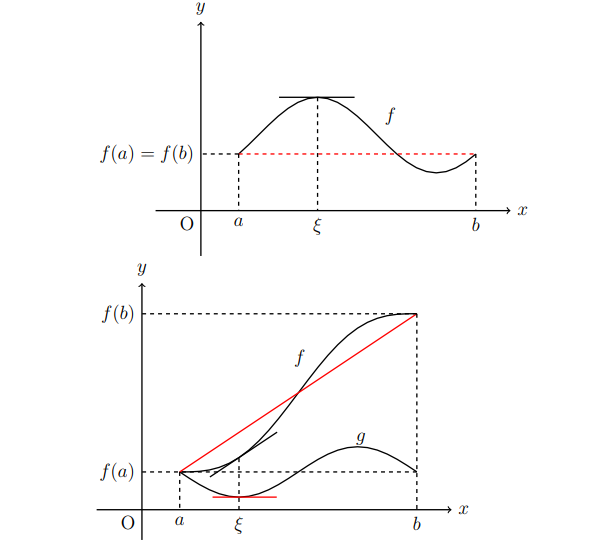代码
\documentclass[a4paper]{article}
\usepackage{pgfplots}
\usepackage{scalefnt}

\pgfplotsset{width=10cm,compat=1.13} % 图片绘制的宽度是7cm,使用的pgfplots版本为1.13

\begin{document}
% 调整字体大小
{\scalefont{1.5}

% 罗尔定理
\begin{tikzpicture}[domain=0:8]
% 画坐标系
\draw[line width=1pt, ->] (-1.2,0) -- (8.2,0) node[right] {$x$};
\draw[line width=1pt, ->] (0,-1.2) -- (0,5) node[above] {$y$};
\draw (0, 0) node[below left] {O};

% sin
\draw[line width=1pt, domain=1:7.28] plot (\x, {2 + sin((\x - 1.5236) r)});
\draw (4.7, 2.5) node[right] {$f$};

% 切线
\draw[line width=1pt] (2.07, 3) -- (4.07, 3);

% 辅助线及各种记号
\draw[line width=1pt, dashed, color=red] (1, 1.5) -- (7.28, 1.5);
\draw[line width=1pt, dashed] (3.09, 3) -- (3.09, 0) node[below] {$\xi$};
\draw[line width=1pt, dashed] (1, 1.5) -- (1, 0) node[below] {$a$};
\draw[line width=1pt, dashed] (7.28, 1.5) -- (7.28, 0) node[below] {$b$};
\draw[line width=1pt, dashed] (1, 1.5) -- (0, 1.5) node[left] {$f(a) = f(b)$};
\end{tikzpicture}

% 拉格朗日中值定理
\begin{tikzpicture}[domain=0:8]
% 画坐标系
\draw[line width=1pt, ->] (-1.2,0) -- (8.2,0) node[right] {$x$};
\draw[line width=1pt, ->] (0,-1.2/1.5) -- (0,9/1.5) node[above] {$y$};
\draw (0, 0) node[below left] {O};

% f = x + sin
\draw[line width=1pt, domain=1:7.28] plot (\x, {(1.5 - sin((\x - 1) r) + (\x - 1))/1.5});
\draw (4.5, 6/1.5) node[left] {$f$};

% g = sin
\draw[line width=1pt, domain=1:7.28] plot (\x, {(1.5 - sin((\x - 1) r))/1.5});
\draw (5.5, 2.8/1.5) node[right] {$g$};

% 切线
\draw[line width=1pt, color=red] (1.87, 0.5/1.5) -- (3.57, 0.5/1.5);
\draw[line width=1pt, domain=1.8:3.58] plot (\x, {(\x - 0.5)/1.5});

% 辅助线及各种记号
\draw[line width=1pt, color=red] (1, 1.5/1.5) -- (7.28, 7.78/1.5);
\draw[line width=1pt, dashed] (2.57, 2.047/1.5) -- (2.57, 0) node[below] {$\xi$};
\draw[line width=1pt, dashed] (7.28, 7.78/1.5) -- (7.28, 0) node[below] {$b$};
\draw[line width=1pt, dashed] (7.28, 7.78/1.5) -- (0, 7.78/1.5) node[left] {$f(b)$};
\draw[line width=1pt, dashed] (1, 1.5/1.5) -- (1, 0) node[below] {$a$};
\draw[line width=1pt, dashed] (7.28, 1.5/1.5) -- (0, 1.5/1.5) node[left] {$f(a)$};
\end{tikzpicture}

}
\end{document}



展开全文• latex画图资料，供学习交流用，教授如何使用latex画图
• Latex提供直接画图的命令,其参考手册是pgf/tikz. 下面给个简单例子: \documentclass[11pt]{article} ... \usepackage{tikz} % Optional PGF libraries \usepackage{pgflibraryarrows} \usepackage{pgflibrarysnakes...

Latex提供直接画图的命令,其参考手册是pgf/tikz.

下面给个简单例子:
\documentclass[11pt]{article}
...
\usepackage{tikz}
% Optional PGF libraries
\usepackage{pgflibraryarrows}
\usepackage{pgflibrarysnakes}
...
\begin{document}
...
\begin{tikzpicture}

\draw (1,0) -- (0,1) -- (-1,0) -- (0,-1) -- cycle;

\end{tikzpicture}
...
\end{document}

参考的文档与工具: 1) http://www.tug.org/TUGboat/tb30-2/tb95mertz.pdf
2) http://www.tug.org/pracjourn/2007-1/mertz/mertz.pdf
3) http://members.multimania.nl/nickvanbeurden/latexpix.htm

直接给出例子的网站: http://www.texample.net/tikz/examples/area/computer-science/

展开全文• LaTex 中，可以直接使用 picture 环境绘制图形。但这种方法具有局限性，比如线段的斜率和圆的半径只能在一个很小的范围内取值。picture 环境提供了 \qbezier 命令，"q" 表示 "quadratic"。许多常用的曲线如圆、...


在 LaTex 中，可以直接使用 picture 环境绘制图形。但这种方法具有局限性，比如线段的斜率和圆的半径只能在一个很小的范围内取值。picture 环境提供了 \qbezier 命令，"q" 表示 "quadratic"。许多常用的曲线如圆、椭圆、或者悬链线都可以用二次 Bezier 曲线得到令人满意的近似，虽然这可能需要一些辛苦的数学准备。虽然在 LaTex 里绘图有局限性而且比较繁琐，但绘图而不插入额外的图片，会使得生成的文档体积较小，比较实用。如果想要在 LaTex 中使用 picture 环境来绘制一些简单的图形，就需要知道它的语法，如下所示：\begin{picture}(x,y)...\end{picture}% 或者\begin{picture}(x,y)(x0,y0)...\end{picture}(x, y) ：在文档中图形保留一个矩阵的区域。(x0, y0) ：可选参数，为矩形左下角指派任意的坐标。相对于 \unitlength 而言，任何时候(除了在picture环境之内以外)，都可以使用命令 \setlength{\unitlength}{1.2cm} 来改变。大多数的绘图命令是下面两种格式之一\put(x,y){object}% 或者\multiput(x,y)(△x,△y){n}{object}(x, y)：起始点。(△x,△y)：从一个对象到下一个的平移向量。{n}：n 为对象的数目。{object}：object 为要绘制的对象。控制绘制图像的线宽的两类命令如下所示：\linethickness{length}：仅对水平和垂直直线及二次 Bézier 曲线有作用。\thinlines 与 \thicklines：可以作用于倾斜的线段、圆和椭圆。\thinlines 让线变得更细；\thicklines 让线变得更粗。下面是使用 \multiput 的案例。\setlength{\unitlength}{2mm}\begin{picture}(30,20)    \linethickness{0.075mm}    \multiput(0,0)(1,0){26}{\line(0,1){20}}    \multiput(0,0)(0,1){21}{\line(1,0){25}}    \linethickness{0.15mm}    \multiput(0,0)(5,0){6}{\line(0,1){20}}    \multiput(0,0)(0,5){5}{\line(1,0){25}}    \linethickness{0.3mm}    \multiput(5,0)(10,0){2}{\line(0,1){20}}    \multiput(0,5)(0,10){2}{\line(1,0){25}}\end{picture}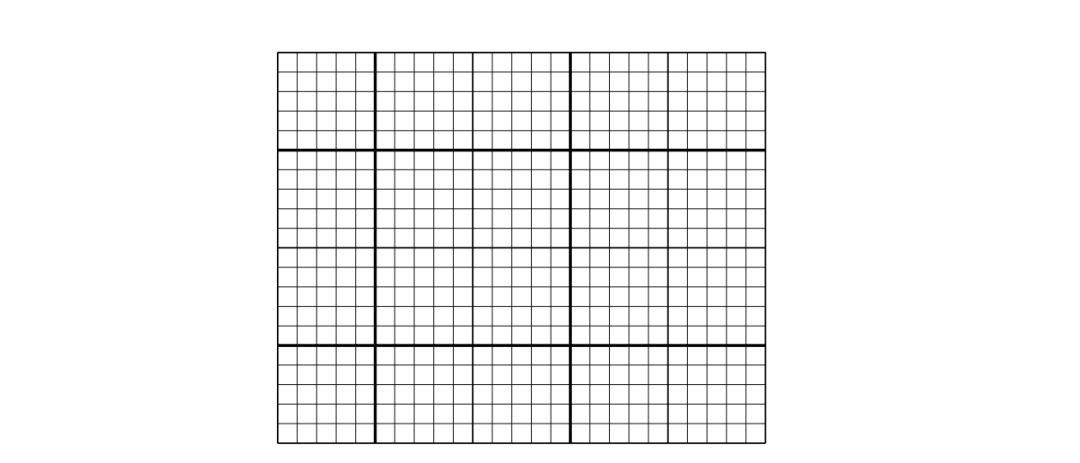圆使用 picture 环境来绘制一个圆，它的语法如下：\put(x,y){\circle{diameter}}% 或\put(x, y){\circle*{diameter}}(x, y) 为圆心坐标。diameter 为圆的直径。picture环境只允许diameter直径最大值约为 14mm。\circle{} 画出空心圆；\circle*{} 画出实心圆。跟线段的情况一样，你可能需要其他宏包的帮助，比如 eepic 或者 pstricks。也可以使用二次 Bézier 曲线拼成一个圆。下面看案例。\setlength{\unitlength}{1mm}\begin{picture}(10,6)    \put(20,30){\circle{1}}    \put(20,30){\circle{4}}    \put(20,30){\circle{8}}    \put(20,30){\circle{14}}\end{picture}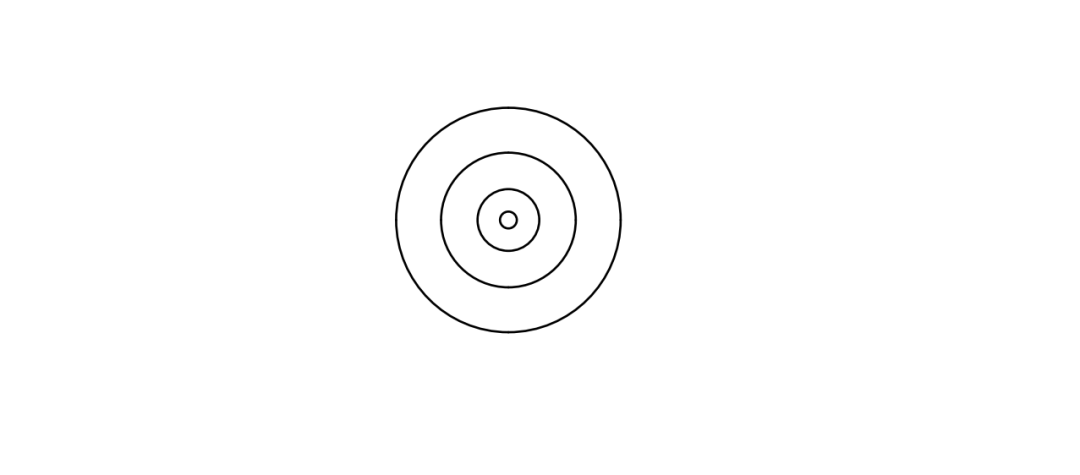\setlength{\unitlength}{1mm}\begin{picture}(10,6)    \put(15,30){\circle*{1}}    \put(20,30){\circle*{4}}    \put(25,30){\circle*{8}}    \put(30,30){\circle*{14}}\end{picture}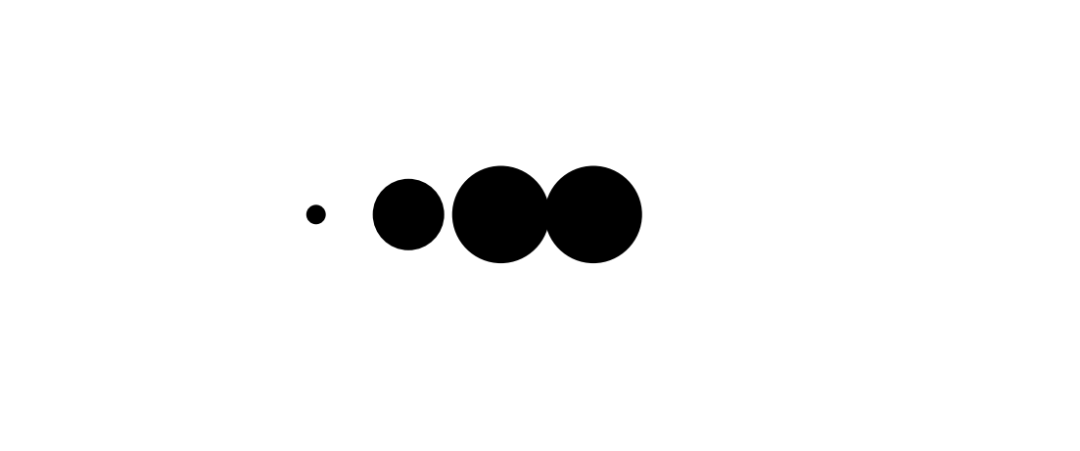椭圆使用 picture 环境绘制椭圆，语法如下：\put(x, y){\oval(w, h)[position]}(x, y) 为椭圆的中心点。(w, h) 中参数 w 表示椭圆的宽，参数 h 表示椭圆的高。[position] 中的参数 position为可选的，它的值可为 b、t、l、r ，分别表示仅绘制椭圆的 “下部”、“上部”、“左部” 和 “右部”。下面看案例。\setlength{\unitlength}{1cm}\begin{picture}(6,6)    \linethickness{0.075mm}    \put(2, 3){\oval(3, 1.8)}\end{picture}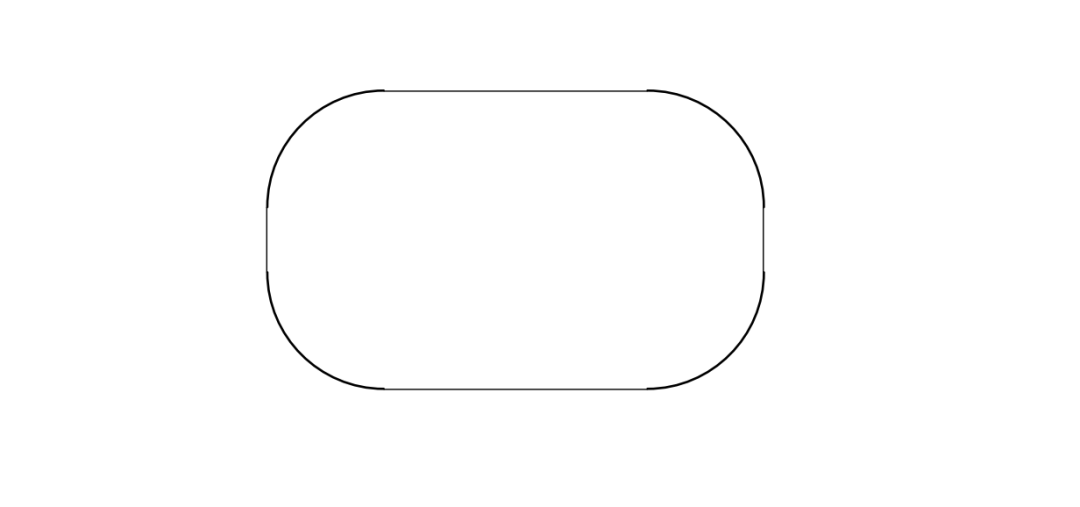\setlength{\unitlength}{1cm}\begin{picture}(6,6)    \thinlines    \put(3, 2){\oval(3, 1.8)}\end{picture}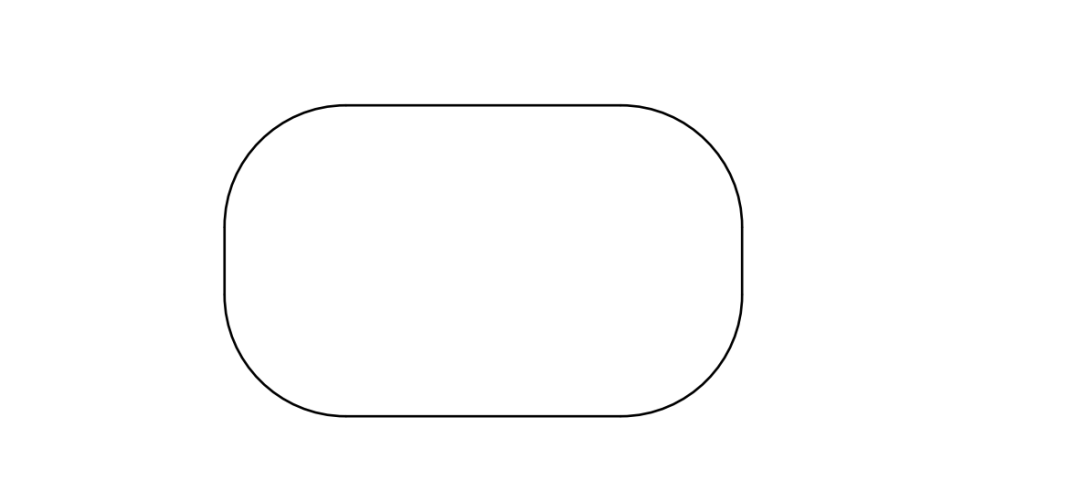\setlength{\unitlength}{1cm}\begin{picture}(6,6)    \thicklines    \put(3, 2){\oval(3, 1.8)}\end{picture}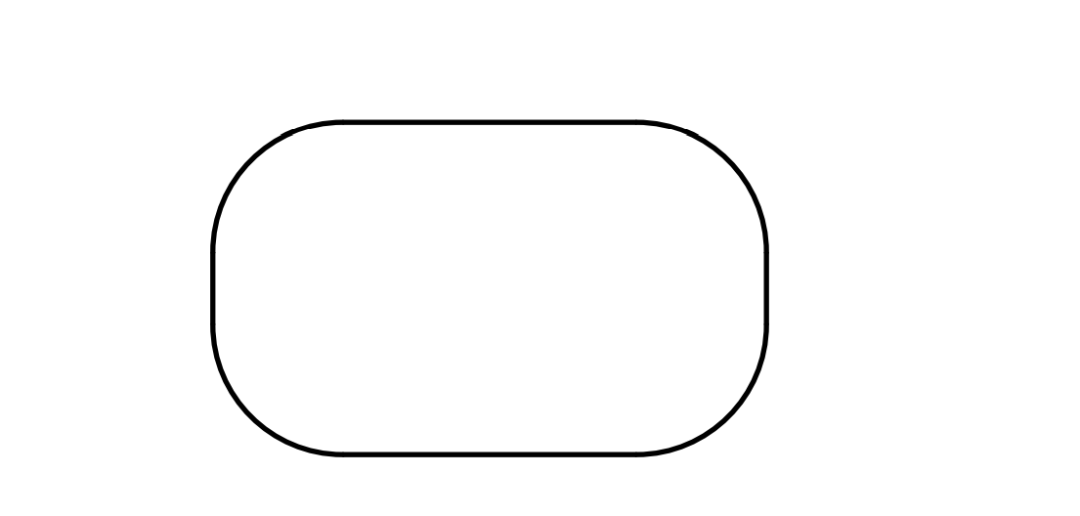可以明显地看出，上面三个图形的线不一样。接着，绘制二分之一椭圆。\setlength{\unitlength}{1cm}\begin{picture}(6,6)    \thicklines    \put(2, 3){\oval(3, 1.8)[b]}\end{picture}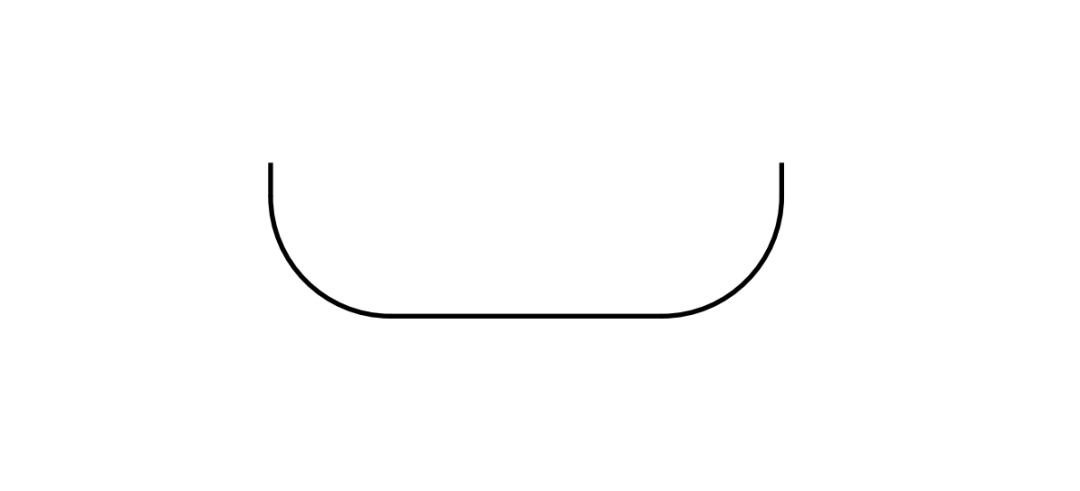下面绘制四分之一椭圆。\setlength{\unitlength}{1cm}\begin{picture}(6,6)    \thicklines    \put(2, 3){\oval(3, 1.8)[bl]}\end{picture}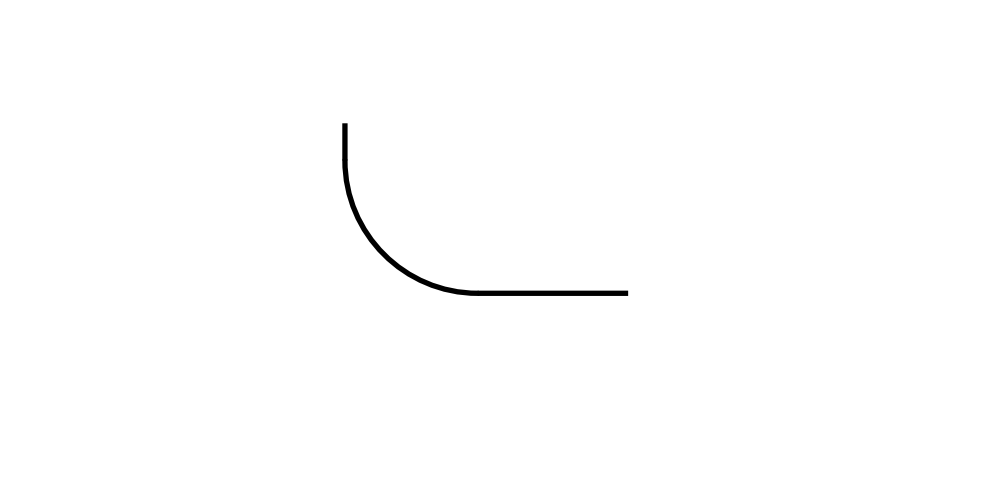线段在 picture 环境中绘制线段，使用如下语法：\put(x,y){\line(x1,y1){length}}(x, y)：线段的绘制起点。(x1, y1)：与(x, y) 相对应的线段方向向量。方向向量需由 -6，-5，...，5，6 整数构成且它们需要互斥(除 1 以外，没有公约数)。[length]：length 表示线段的长度。长度是相对于 \unitlength 来说的。下面看案例。\setlength{\unitlength}{5cm}\begin{picture}(1,1)    \put(0, 0){\line(1, 0){1}}    \put(0,0){\line(1,1){.2}}    \put(.2,.2){\line(2,1){.33}}    \put(.53, .365){\line(5,1){.15}}    \put(0, 0){\line(0, 1){1}}\end{picture}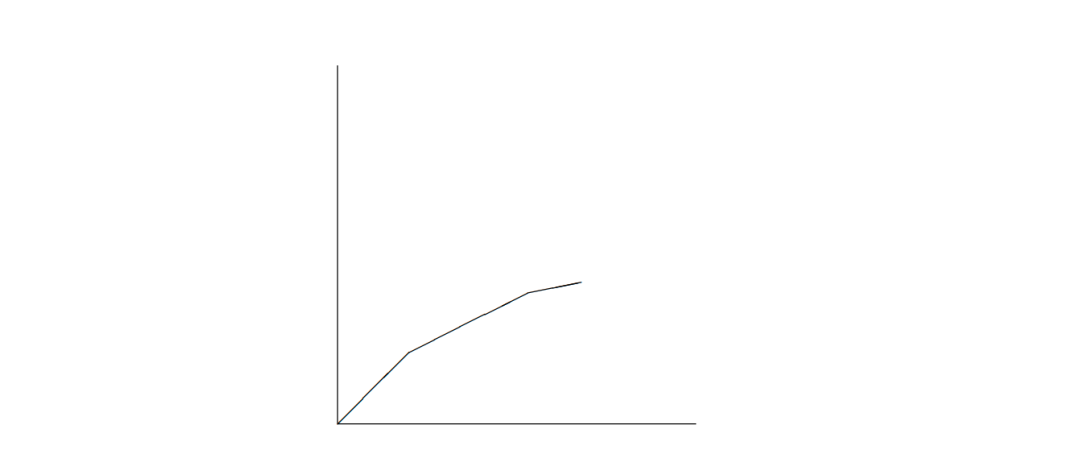箭头在 picture 环境中绘制箭头，使用如下语法：\put(x,y){\vector(x1,y1){length}}(x, y)：箭头绘制起点。(x1, y1)：与 (x, y) 相对的箭头方向向量。方向向量需由 -4，-3，...，3，4 整数构成且它们需要互斥(除 1 以外，没有公约数)。[length]：length 表示线段长度。注意：命令 \thicklines 对指向左上方的两个箭头产生效果。下面看案例。\setlength{\unitlength}{0.75mm}\begin{picture}(1,1)    \put(0, 0){\vector(1, 0){30}}    \put(0, 0){\vector(4, 1){20}}    \thicklines    \put(0, 0){\vector(-4, 1){25}}    \thinlines    \put(0, 0){\vector(-1, -4){5}}\end{picture}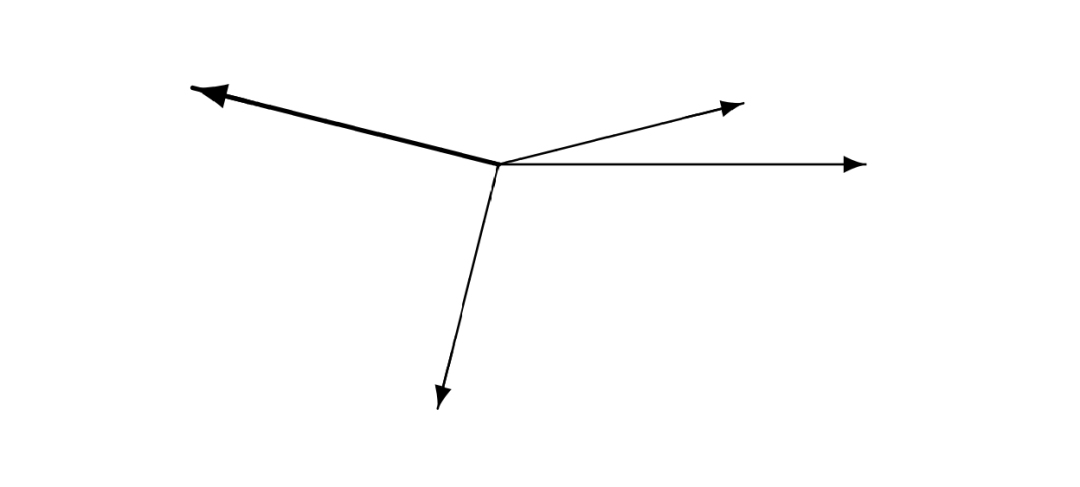公式在 picture 环境中使用 \put 命令绘制文本与公式。如下所示：\setlength{\unitlength}{0.8cm}\begin{picture}(6,5)    \thicklines    \put(1,0.5){\line(2,1){3}}    \put(4,2){\line(-2,1){2}}    \put(2,3){\line(-2,-5){1}}    \put(0.7,0.3){$A$}    \put(4.05,1.9){$B$}    \put(1.7,2.95){$C$}    \put(3.1,2.5){$a$}    \put(1.3,1.7){$b$}    \put(2.5,1.05){$c$}    \put(0.3,4){$F=\sqrt{s(s-a)(s-b)(s-c)}$}    \put(3.5,0.4){$\displaystyle s:=\frac{a+b+c}{2}$}\end{picture}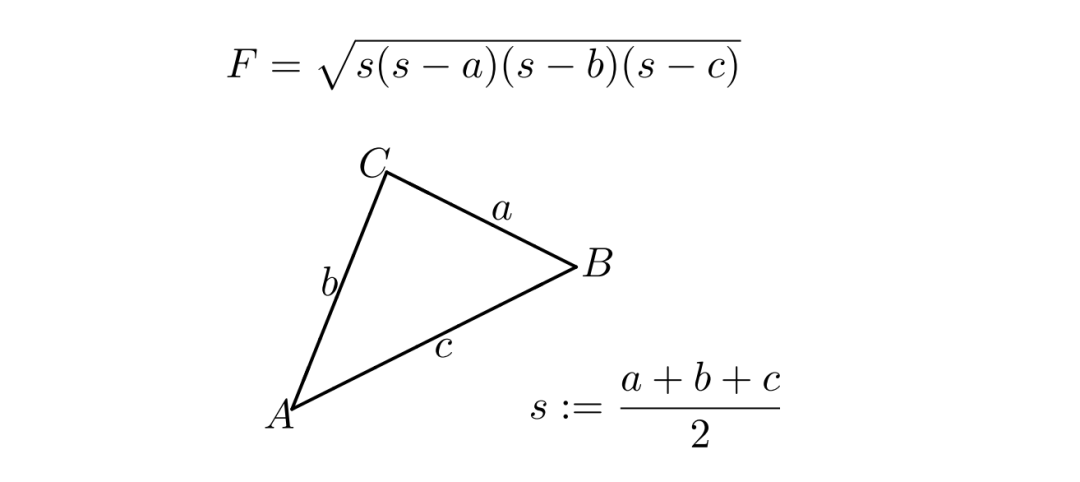二次 Bézier 曲线Bézier 曲线需要如下命令绘制：\qbezier(x1,y1)(x,y)(x2,y2)(x1, y2)：Bézier 曲线中的起点。(x, y)：Bézier 曲线的中间控制点。(x2, y2)：Bézier 曲线中的终点。令 P1=(x1,y1)，P2=(x2,y2)和 m1，m2分别表示一条二次 Bézier 曲线的两个端点及其对应斜率。中间控制点 S = (x, y) 则由下述方程给出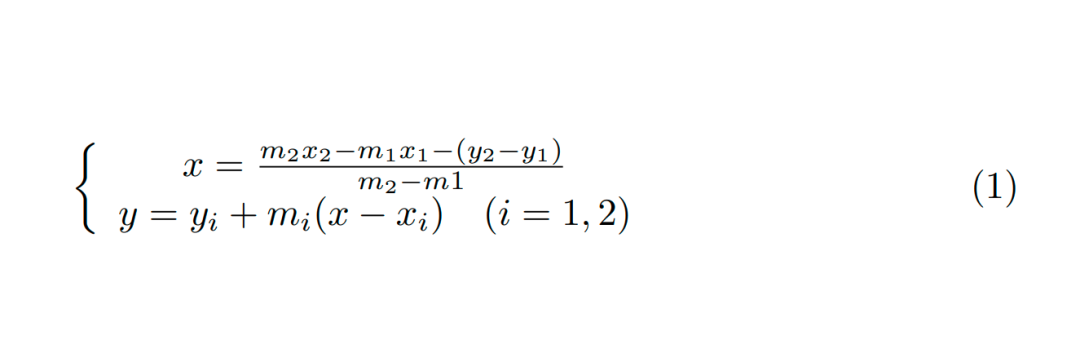下面是使用二次 Bézier 曲线拼接的圆形。\setlength{\unitlength}{0.8cm}\begin{picture}(6,4)    \qbezier(4,2)(4,3)(3,3)    \qbezier(3,3)(2,3)(2,2)    \qbezier(2,2)(2,1)(3,1)    \qbezier(3,1)(4,1)(4,2)\end{picture}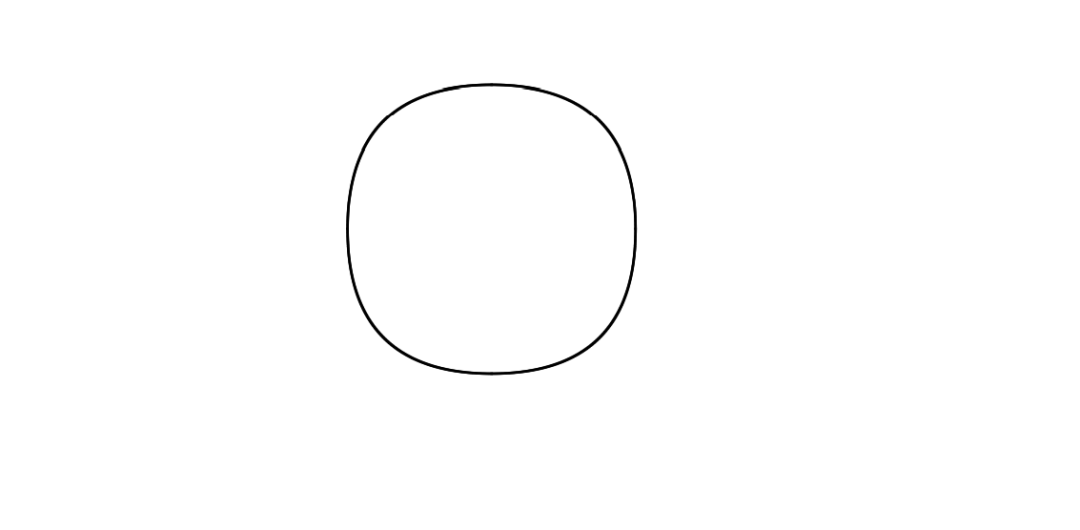使用四条二次 Bézier 曲线拼成圆形的效果不是很让人满意。再看一下其他的案例。\setlength{\unitlength}{0.8cm}\begin{picture}(6,4)    \put(0.5,0.5){\line(1,5){0.5}}    \put(1,3){\line(4,1){2}}    \qbezier(0.5,0.5)(1,3)(3,3.5)\end{picture}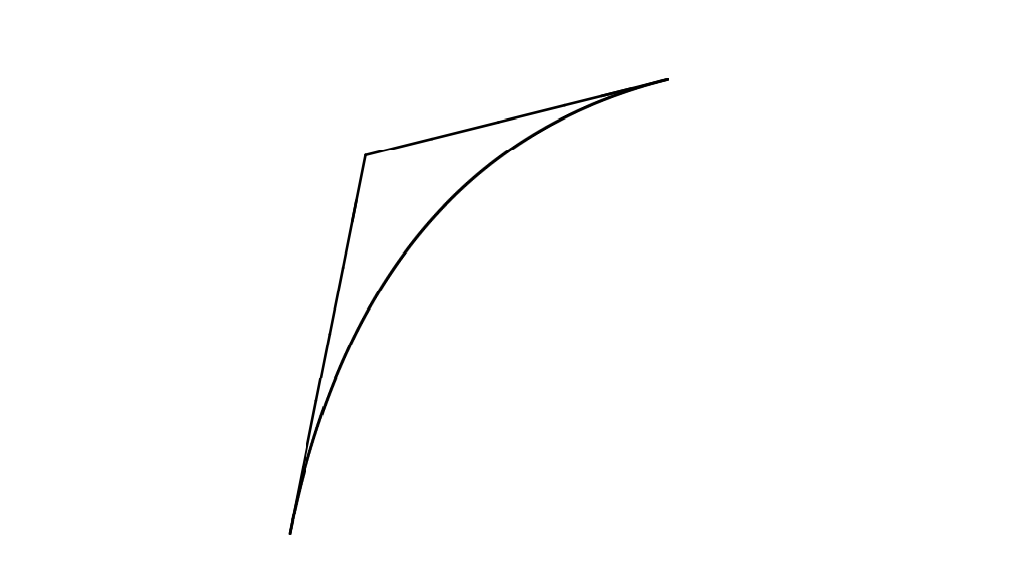\setlength{\unitlength}{0.8cm}\begin{picture}(6,4)    \thinlines    \put(2.5,2){\line(2,-1){3}}    \put(5.5,0.5){\line(-1,5){0.5}}    \linethickness{1mm}    \qbezier(2.5,2)(5.5,0.5)(5,3)\end{picture}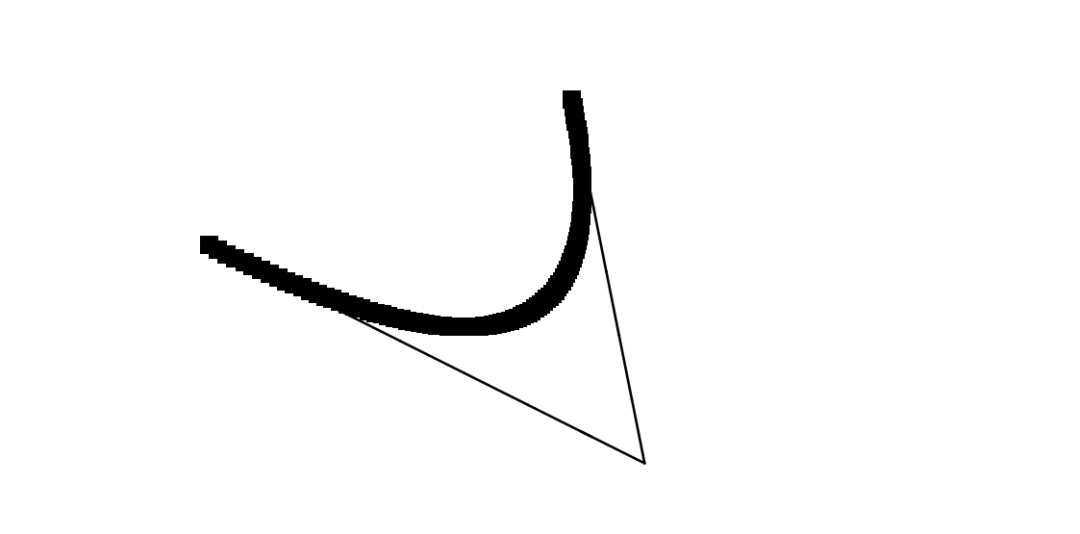悬链线悬链线指的是两端固定的一条均匀、柔软的链条，在重力的作用下所具有的曲线形状。下面画出一条悬链线。\setlength{\unitlength}{1cm}\begin {picture} (4.3, 3.6)(-2.5,-0.25)    \put(-2,0){\vector(1,0){4.4}}    \put(2.45,-.05){$x$}    \put(0,0){\vector(0,1){3.2}}    \put(0,3.35){\makebox(0,0){$y$}}    \qbezier(0.0,0.0)(1.2384,0.0)(2.0,2.7622)    \qbezier(0.0,0.0)(-1.2384,0.0)(-2.0,2.7622)    \linethickness{.075mm}    \multiput(-2,0)(1,0){5}{\line(0,1){3}}    \multiput(-2,0)(0,1){4}{\line(1,0){4}}    \linethickness{.2mm}    \put( .3,.12763){\line(1,0){.4}}    \put(.5,-.07237){\line(0,1){.4}}    \put(-.7,.12763){\line(1,0){.4}}    \put(-.5,-.07237){\line(0,1){.4}}    \put(.8,.54308){\line(1,0){.4}}    \put(1,.34308){\line(0,1){.4}}    \put(-1.2,.54308){\line(1,0){.4}}    \put(-1,.34308){\line(0,1){.4}}    \put(1.3,1.35241){\line(1,0){.4}}    \put(1.5,1.15241){\line(0,1){.4}}    \put(-1.7,1.35241){\line(1,0){.4}}    \put(-1.5,1.15241){\line(0,1){.4}}    \put(-2.5,-0.25){\circle*{0.2}}\end{picture}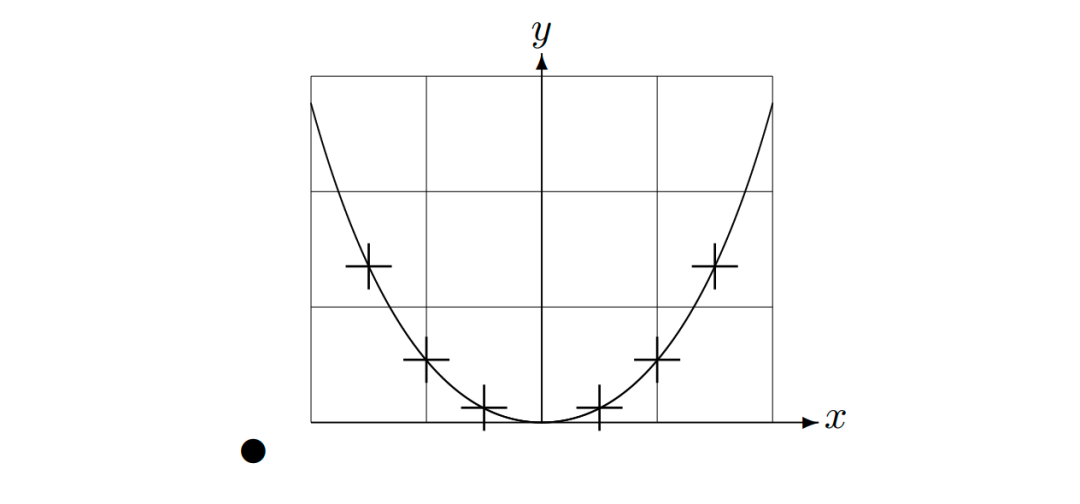在上图中，悬链线 y=cosh x - 1 对称的两半由二次 Bézier 曲线分别近似地绘成。曲线的右半部分终止于点 (2, 2.7622)，对应的斜率为 m=3.6269。再次使用 Bézier 的公式，可以计算中间控制点。计算结果为 (1.2384, 0) 和 (-1.2384, 0)。图中的十字为真正的悬链线上的点。误差小于百分之一，很难被发现。该例指出了命令 \begin{picture} 的可选参数的用法。该图通过使用命令\begin{picture}(4.3, 3.6)(-2.5, -0.25)定义了方便的 "数学" 坐标：左下角(由黑色圆点标出)坐标是 (-2.5, -0.25)。坐标的相对性Bezier的公式给出了两条 Bezier 曲线的控制点。正向分支由 P1=(0,0),m1=1和 P2=(2,tanh2),m2=1/cosh22确定。与前例相同，本图也定义了在数学上方便的坐标，左下角的坐标是 (-3, -2)(黑点)。下面看案例。\setlength{\unitlength}{0.8cm}\begin{picture}(6,4)(-3,-2)    \put(-2.5,0){\vector(1,0){5}}    \put(2.7,-0.1){$\chi$}    \put(0,-1.5){\vector(0,1){3}}    \multiput(-2.5,1)(0.4,0){13}{\line(1,0){0.2}}    \multiput(-2.5,-1)(0.4,0){13}{\line(1,0){0.2}}    \put(0.2,1.4){$\beta=v/c=\tanh\chi$}    \qbezier(0,0)(0.8853,0.8853)(2,0.9640)    \qbezier(0,0)(-0.8853,-0.8853)(-2,-0.9640)    \put(-3,-2){\circle*{0.2}}\end{picture}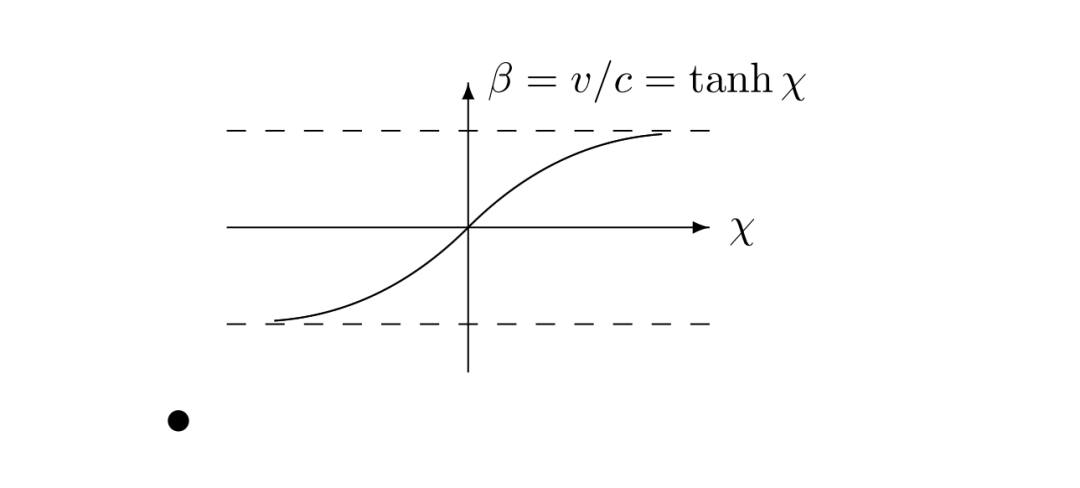预定义的图形盒子在 picture 环境中绘制图形盒子，使用如下语法进行声明：\newsavebox{name}声明后，使用如下语法进行定义：\savebox{name}{width, height}[position]{content}最后一步，使用如下语法重复绘制：\put(x, y)\usebox{name}{name}：参数name 指明了 LaTex 存储槽，揭示了其命令本质。{position}：可选参数 position，用于定义图形存放盒子的 “锚点”。t、l、b、r 分别描述的位置为 “上”、“左”、“下”、“右”。\setlength{\unitlength}{0.5mm}\begin{picture}(120,168)    \newsavebox{\foldera}    \savebox{\foldera}(40,32)[bl]{% definition        \multiput(0,0)(0,28){2}{\line(1,0){40}}        \multiput(0,0)(40,0){2}{\line(0,1){28}}        \put(1,28){\oval(2,2)[tl]}        \put(1,29){\line(1,0){5}}        \put(9,29){\oval(6,6)[tl]}        \put(9,32){\line(1,0){8}}        \put(17,29){\oval(6,6)[tr]}        \put(20,29){\line(1,0){19}}        \put(39,28){\oval(2,2)[tr]}    }    \newsavebox{\folderb}    \savebox{\folderb}(40,32)[l]{% definition        \put(0,14){\line(1,0){8}}        \put(8,0){\usebox{\foldera}}    }    \put(34,26){\line(0,1){102}}    \put(14,128){\usebox{\foldera}}    \multiput(34,86)(0,-37){3}{\usebox{\folderb}}\end{picture}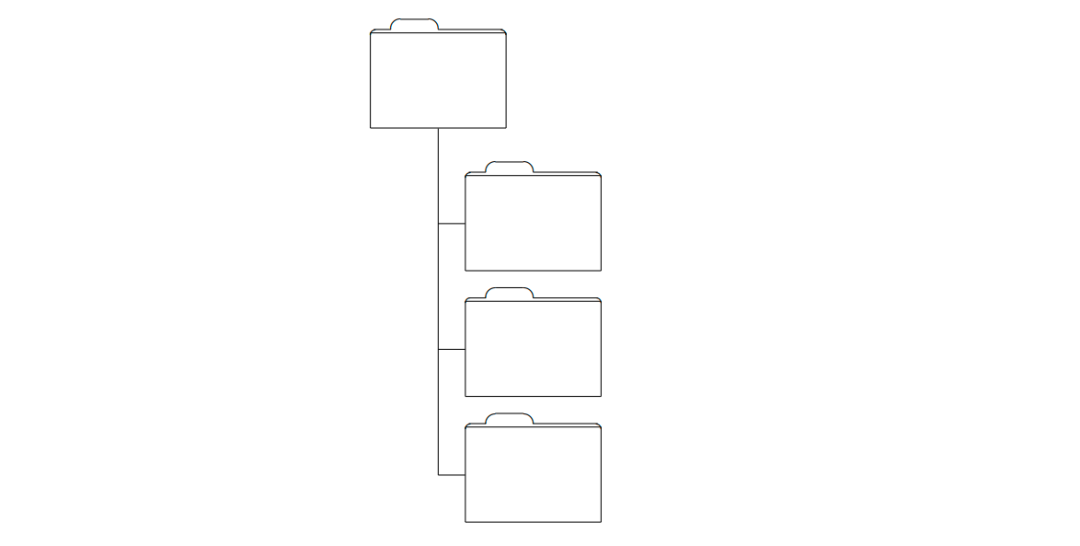由于命令 \line 在线段长度小于大约 3mm 的时候不能正常工作，所以必须使用命令 \oval。总结在 LaTex 中，使用 picture 环境绘制图形有较大的局限性且比较繁琐，但也有其优点，就是不需要插入额外的图片。这篇文章主要是介绍了使用 picture 环境简单的绘制圆、椭圆、线段、箭头、Bézier曲线等图形。参考资料一份不太简短的LATEX2介绍 作者：Tobias Oetiker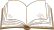你的赞和在看，我都喜欢！

展开全文• 虚线，实线，双向箭头，箭头粗细，等等，都是在画图过程中，会遇到的问题，下面用一个简单案例展示，如何设计箭头，线条等 \begin{tikzpicture}[auto, box/.style = {draw, minimum height=8cm, minimum width=3...
虚线，实线，双向箭头，箭头粗细，等等，都是在画图过程中，会遇到的问题，下面用一个简单案例展示，如何设计箭头，线条等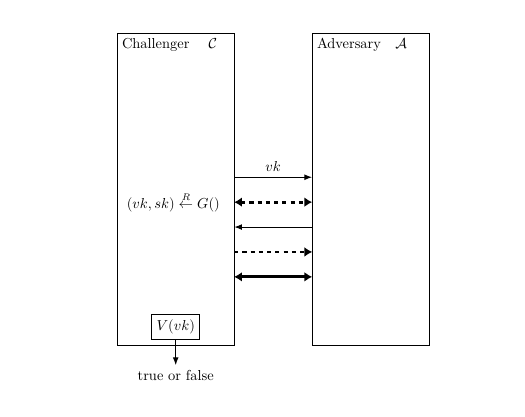\begin{tikzpicture}[auto,
box/.style = {draw, minimum height=8cm, minimum width=3cm, align=left, outer sep=0pt},
lbl/.style = {anchor=north west, outer sep=0mm},
arrow1/.style = {
draw = black, thick, -{Latex[length = 2mm, width = 1.5mm]},
},
arrow2/.style = {
draw = black, dashed, line width=2pt, {Latex[length = 2mm, width = 2.5mm]}-{Latex[length = 2mm, width = 2.5mm]},
},
arrow3/.style = {
draw = black, line width=2pt, {Latex[length = 2mm, width = 2.5mm]}-{Latex[length = 2mm, width = 2.5mm]},
},
arrow4/.style = {
draw = black, dashed, line width=1pt, -{Latex[length = 2mm, width = 2.5mm]},
} ]

\node (n1)  [box,
label={[lbl] north west:Challenger ~~ $\mathcal{C}$}
]	{\begin{aligned}\\ (vk,sk) \overset{R}{\leftarrow} G() \end{aligned}	};

\node (n3)  [box,
label={[lbl]north west:Adversary~~ $\mathcal{A}$},
right=20mm of n1
]
{	};

\node (n5) [draw,below=-8mm of n1]{$V(vk)$};
\node (n4) [below=5mm of n1]{true or false};

\path[arrow1] ([yshift=2ex]  n1.east) -- node{$vk$} ([yshift=2ex] n3.west);
\path[arrow2] ([yshift=-2ex]  n1.east) -- ([yshift=-2ex] n3.west);
\path[arrow4] ([yshift=-10ex]  n1.east) -- ([yshift=-10ex] n3.west);
\path[arrow1] ([yshift=-6ex] n3.west) --  ([yshift=-6ex] n1.east);
\path[arrow3] ([yshift=-14ex] n3.west) -- ([yshift=-14ex] n1.east);
\path[arrow1] (n5) -- (n4);
\end{tikzpicture}

在导言区添加几行代码

\usepackage{tikz}
\RequirePackage{amsmath,mathrsfs,amsfonts,amssymb}


上面的代码能够实现图中所示的案例，关于线条的更多控制，补充下面一些内容，各个属性可以组合使用，如案例所示

线条粗细控制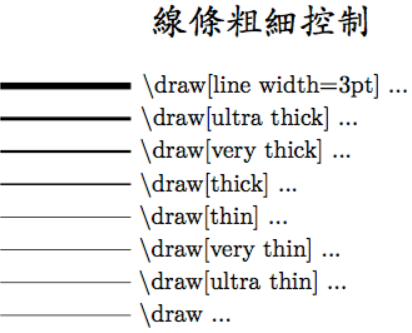线条颜色，透明度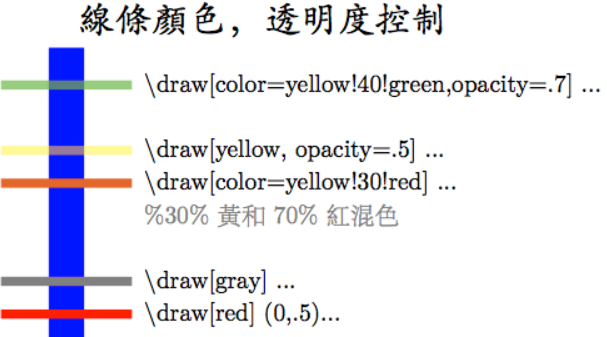线条样式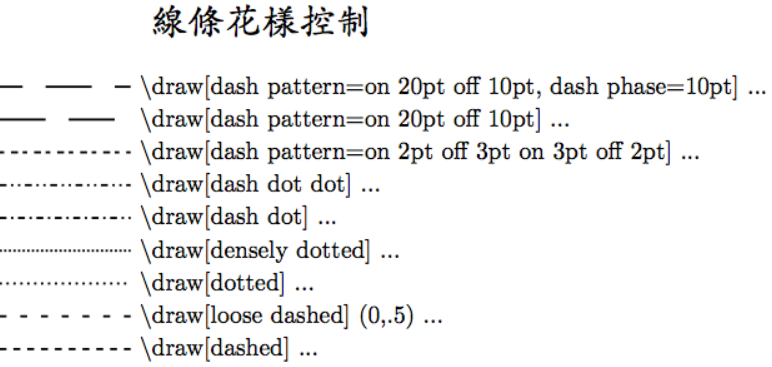线条两端控制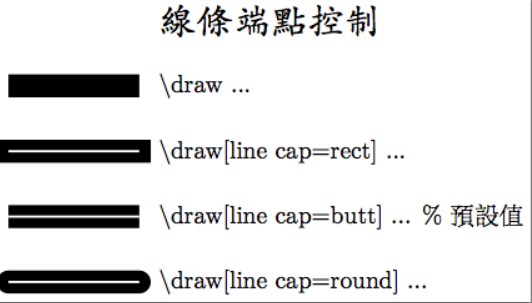折线控制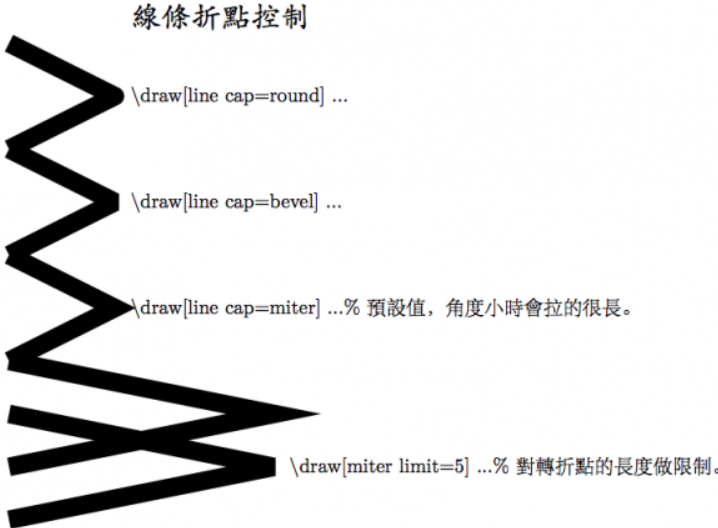展开全文• 2）打开.pdf格式的图片，发现图片只占用了整个页面的一部分，如果直接将此图片转换成.eps格式的图片，将其插入到Latex之后，会有很大的空白出现。因此需要将图片剪裁成合适的尺寸。我的方法是将.pdf的图片打开，然后...visio
• 【收录队友的论文博客】正是由于数学建模美赛，我们队伍的同学才开始去学习...于是我们有个西电的保研群里面就开始天天问“字体用什么”“标题间距”“怎么调画图”等等之类的问题而如果你学会了Latex这样一个工具...
•  写论文的时候经常使用Visio来画图，然而用Latex排版论文的话，只能插入.eps格式的图片文件。这篇文章举个简单例子，说明一下这个过程。  1、使用Visio画图 按照过程：文件->另存为->保存类型选择（PDF*.pdf）->...
• 写论文的时候经常应用Visio来绘图，然而用Latex排版论文的话，只能插入.eps格式的图片文件。这篇文章举个简单例子，说明一下这个过程。 1、应用Visio绘图 每日一道理 “上下五千年，龙的看火不灭；古有愚公志，...
• 此文讲解如何向Matlab图形中添加Latex公式说明。 我们首先画一个正弦图形，代码如下： x = 0:0.01:(2*pi); y = sin(x); plot(x,y,'k'); 执行上面的代码，会得到如下所示的图形。 接下来...
• 如图所示，在上面有一个矩形框，下面两个矩形框，如何让上面矩形框居中，下面矩形框也居中？ 采用一种比较讨巧的方式实现：在上面矩形框的下面定义一个无内容的空矩形框，然后下面两个矩形框在这个空矩形框的基础上...居中对齐
• 由以下代码生成的轴刻度标记标签的字体不是Helvetica，但仍然是默认的衬线计算机Modern。任何建议都非常感谢。from matplotlib import rc, font_managerfrom numpy import arange, cos, pifrom matplotlib.pyplot ...
• 我的代码：\begin{figure}[H]\begin{center}\begin{tikzpicture}[->,>=stealth',auto,nodedistance=7em,thick,mainnode/.style={circle,fill=blue!2,draw,font=\sffamily\small}]%fon...我的代码：\begin{...
• 情况一：visio画图 1. visio 另存为pdf (如果想要高像素图，visio保存为svg，然后利用AI另存为pdf) 2. Adobe Acrobat 9 Pro 去空白边框 另存为eps 情况二：matlab画图 1. plot画图 调整好大小 2. 另存...
• 如何latex 中插入EPS格式图片 第一步：生成.eps格式的图片 1、利用visio画图，另存为pdf格式的图片 利用Adobe Acrobat裁边，使图片大小合适 另存为.eps格式，如下图所示： 注：这一步必须按照图示方式...
• 使使用用python matplotlib 画画图图导导入入到到word 中中如如何何保保证证分分辨辨率率 这篇文章主要介绍了使用python matplotlib 画图导入到word中如何保证分辨率的例子具有很好的参考价值希 对大家有所帮助一起...
• 在写论文时，如果是菜鸟级别，可能不会花太多时间去学latex，直接用word去写，但是这有一个问题，当我们用其他工具画完实验彩色图时，放到word中会有比较模糊，这有两个原因导致的。原因一：图片导入word中，word会...
• Visio 画图是很正常的，转化为EPS则较为麻烦，现提供一条可行路径 保存为PDF，在利用Photoshop转化为EPS。 我也是醉了。。 转载于:https://www.cnblogs.com/yuanqian-PM/p/4213672.html...
• 在写论文时，如果是菜鸟级别，可能不会花太多时间去学latex，直接用word去写，但是这有一个问题，当我们用其他工具画完实验彩色图时，放到word中会有比较模糊，这有两个原因导致的。原因一：图片导入word中，word会...
• 不必使用图形软件画图、存盘并插 入 L ATEX 文档，你可以直接描述图形，然后 L ATEX 会替你画好它。 第六章 包含一些潜在的危险信息，内容是关于如何改变 L ATEX 所产生文档的标 准布局。它会告诉你如何把 L ATEX 的...
• 在写论文时，如果是菜鸟级别，可能不会花太多时间去学latex，直接用word去写，但是这有一个问题，当我们用其他工具画完实验彩色图时，放到word中会有比较模糊，这有两个原因导致的。 原因一：图片导入word中，word会...
• 阅读这一章后，你应该对LATEX 如何工作有一个大致的理解。 第二章探究文档排版的细节。它解释了大部分必要的LATEX 命令和环境。在阅 读完这一章之后，你就能够编写你的第一份文档了。 第三章解释了如何使用LATEX ...
• 如何将SmartDraw中的图形导出LATEX可用的EPS格式？ 下面介绍两种可行的方法，自己弄了一下午总结出来的经验，希望能帮到遇到问题的朋友： 1. 用smartdraw画图，用save as 存成eps文件，在转化过程中只选择include AI...include
• 具体做法如下：　使用WinFig或者Xfig来画图，在输入Text时，将右方special框按下，按照LaTeX输入公式的方法来输入，例如$S_3$等等。保存时按照默认方式保持为pxtex文件，保存后产生两个文件.pxtex和.pxt